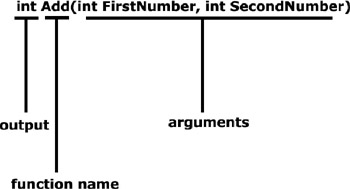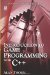# 9.2 Functions in C

## 9.2 Functions in C++

Functions in C++ are more or less analogous to functions in mathematics. Functions in C++ are like processing machines; they can output one value, and they can also accept one or more arguments as inputs. In C++ the inputs and outputs are not restricted to just numbers; output and input values can be chars, floats, Booleans, or any variable data type.

Furthermore, functions are a good way of dividing code into smaller, more manageable blocks that can be reused. Thus far in this book, only one function has been considered in C++-the main function, where a program begins and ends. Now that we understand functions a bit, we can begin coding our own functions.

### 9.2.1 Coding Functions

Functions take the following form:

`      DataType FunctionName (Argument1, Argument2, ...)      {         do something;         return Output;      } `

A function can be thought of as a section of code that is identified by a name; it can accept arguments as input and can return a value as output. In C++, the output value is called a return value, and the inputs are called arguments. A function can accept none, one, or many arguments, but can have only one return value. Coding a function in C++ is based on the standard mathematical function notation. Consider the following code:

`      int Add(int FirstNumber, int SecondNumber)      {         int Result = 0;         Result = FirstNumber + SecondNumber;         return Result;      } `
 Note I have written separate lines for clarity, but the above function can be abbreviated to: ` int Add(int FirstNumber, int SecondNumber) { return (FirstNumber + SecondNumber); } `

Let's examine the function declaration:Figure 9.1

The first part, int, specifies the data type of the value to be returned; in this case, the result of adding the two arguments. Any data type can be used as an output value. Next appears the function name, Add; as with variables, this can be any name a programmer chooses. The parentheses enclose the arguments of the function. Empty parentheses indicate the function has no arguments. The example has two integer arguments, although any data type can be used.

Next, the code inside the braces ({}) is the body of the function, also called the function definition. This is the code that will be executed each time the function is instructed to run. The return keyword is used to output a value whose data type matches the return type, which in this case is int. The return keyword will also bring about an end to the function. The function will exit when this keyword is encountered, so any lines written after this inside the function will be ignored by the compiler.

### 9.2.2 Calling Functions

The following sample program contains two functions: the required main function and the add function. As the application is executed, it begins at the top of main (not add). It therefore prints "hello world" and then terminates. The add function is not executed.

`      #include <iostream>      int Add(int FirstNumber, int SecondNumber)      {         return (FirstNumber + SecondNumber);      }      int main()      {         std::cout<<"hello world\n";         return 0;      } `

In order for the add function to be executed it needs to be called. This means we need to tell the compiler it should be executed. The add function requires two arguments: the two integers to add. Functions are called by name, as follows:

`      #include <iostream>      int Add(int FirstNumber, int SecondNumber)      {         return (FirstNumber + SecondNumber);      }      int main()      {         int Number1 = 0;         int Number2 = 0;         std::cout<<"Enter First Number\n";         std::cin >> Number1;         std::cout<<"Enter Second Number\n";         std::cin >> Number2;         int Result = Add(Number1, Number2);         std::cout<<"Result is "<<Result;         return 0;      } `
 Note Result is used to store the output of the function add, and two integers are provided as inputs to add.Introduction to Game Programming with C++ (Wordware Game Developers Library)
ISBN: 1598220322
EAN: 2147483647
Year: 2007
Pages: 225
Authors: Alan Thorn

Similar book on Amazon

flylib.com © 2008-2017.
If you may any questions please contact us: flylib@qtcs.net